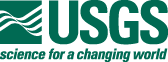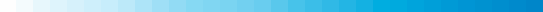New England Aeromagnetic Data ProcessingThe assembly of the 80 individual aeromagnetic surveys and grids to create the New England compilation was done in several steps.

DATA PROCESSING STEPS

1. Grids were constructed from the original aeromagnetic survey data, projected to the specifications given below, with a cell size of between 1/3 and 1/5 of the flight-line spacing of the survey, using a minimum curvature gridding algorithm. For digitized contour line data, the initial grid was constructed using a minimum curvature algorithm and a spacing appropriate for the scale of the digitized map.
2. Where feasible, the Definitive Geomagnetic Reference Field (DGRF) was calculated for the date and altitude of the original survey. Where only residual field field data are available, the original reference field may be added back if the field specifics are known; then the DGRF is removed.
3. Flight lines were adjusted and spurious anomalies (generated by equipment malfunction) were removed as necessary.
4. Strike filtering in the direction of the flight lines was applied as necessary.
5. The survey grids were regridded, as necessary, to the final grid cell size of 500 m using a minimum curvature algorithm.
6. The original survey grids were continued to 1000 ft above ground and converted from level to drape as necessary. Upward continuation of surveys flown at elevations less than 1000 ft above ground was by standard 2D Fast Fourier Transform (FFT) filtering techniques. Surveys flown at altitudes greater than 1000 ft were continued downward by means of the "Compudrape" program in the Oasis Montaj package by Geosoft.
7. The datum levels of the converted grids were adjusted (by addition or subtraction of a constant value) to minimize differences at the boundaries.
8. These grids were combined into a single merged grid that shows the magnetic field measured or calculated at 1000 ft above ground. This grid is available for downloading in several formats.

GRID PROJECTION SPECIFICATIONS

• Projection = Lambert conformal conic
• Central meridian = 74 degrees W
• Base latitude = 0 degrees N
• Standard parallels = 33 and 45 N
• Semi-major ellipsoid axis = 6378206.4 m
• Eccentricity squared = 0.0067686579973